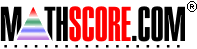Math Practice Online > free > lessons > Florida > 6th grade > Congruent And Similar Triangles

## Congruent And Similar Triangles

This topic deals with recognizing congruent and similar triangles.

 Sample Problems for Congruent And Similar Triangles Lesson for Congruent And Similar Triangles

### This topic aligns to the following state standards

Grade 2: Geo 3. Knows congruent shapes.
Grade 3: Geo 3. Knows congruent and similar figures.
Grade 4: Geo 3. Knows and creates congruent and similar figures.
Grade 5: Geo 3. Knows how to justify that two figures are similar or congruent.
Grade 6: Geo 3. Recognizes and draws congruent and similar figures.
Grade 7: Geo 3. Recognizes, draws, and describes congruent and similar figures.

Copyright Accurate Learning Systems Corporation 2008.